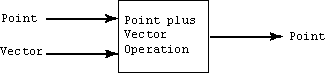Add the vector (1, 2)T to the point (4, 4)T.

The result is a point: (4, 4)T + (1, 2)T = ( 5, 6 )T

# Adding Points and Vectors

If the vector (1, 2)T is a displacement (i.e., an amount by which to change x, and an amount by which to change y), then the result must be a point in a new location:

```(4, 4)T +  (1, 2)T  = ( 5, 6 )T
```

This is one of those situations where using the same representation (i.e. column matrices) for both points and vectors is confusing. You have to keep track of what type of object each matrix represents.

A displacement vector added to a point results in a point. Here is a diagram of that:This is slightly odd. Two mathematical objects of different types are added together. Again, the "+" sign is overloaded.

### QUESTION 20:

Continue to the next page.